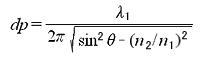# Q: How deep does the infrared light penetrate at the position of contact between the prism and sample during ATR measurements?

A : The following factors contribute to the depth of light penetration for ATR measurements: (1) Wavenumber, (2) angle of incidence, and (3) refractive index. Based on these factors, the depth of penetration into the sample at the contact position is given by the following equation.(1) Wavenumber
From the equation above, the penetration depth increases at lower wavenumbers (longer wavelengths). As shown in the diagrams below, the peak intensities at the low-wavenumber side of the ATR spectrum are much higher than in the transmission spectrum.

(2) Angle of incidence
The penetration depth decreases as the angle of incidence increases.

(3) Refractive index
If the same sample is measured using prisms of different refractive index, the depth of penetration is lowest for the prism with the highest refractive index. The depth of light penetration can be theoretically calculated using the equation above.

Theoretically Calculated Depth of Light Penetration

 Wavenumber/cm-1 Prism (refractive index) ZnSe or KRS-5 (2.4) Ge (4.0) 4000 0.5 µm 0.17 µm 3000 0.66 µm 0.22 µm 2000 1.01 µm 0.33 µm 1500 1.35 µm 0.44 µm 1000 2.01 µm 0.66 µm 400 5.03 µm 1.66 µm

A 45° angle of incidence and the sample refractive index of a general organic compound (n2 = 1.50) were used for the theoretical calculations. Therefore, the peaks obtained may differ according to the prism selected when a multilayer film is measured.